# RANSAC Robust model estimation from data contaminated by

• Slides: 17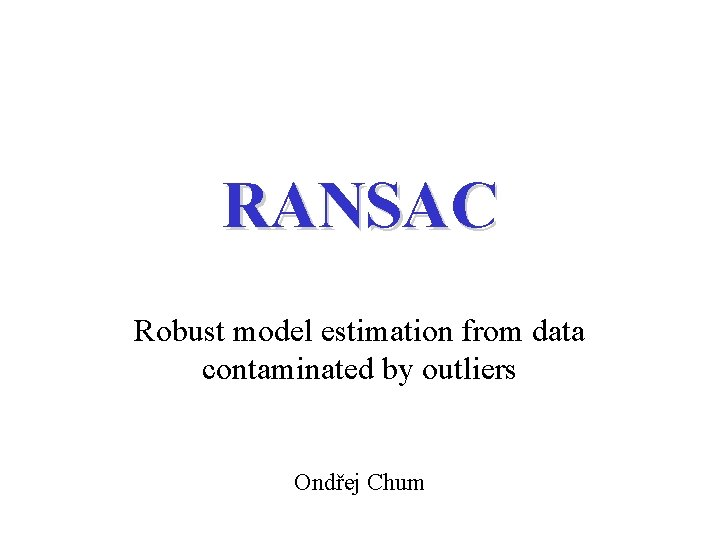RANSAC Robust model estimation from data contaminated by outliers Ondřej ChumFitting a Line Least squares fit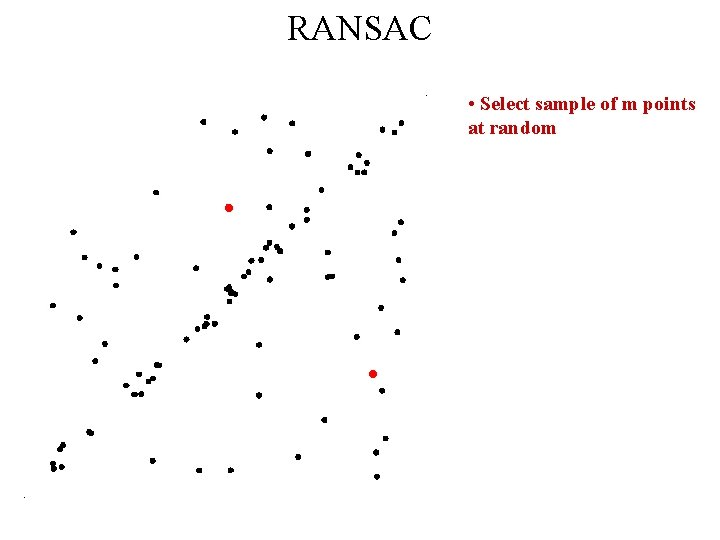RANSAC • Select sample of m points at randomRANSAC • Select sample of m points at random • Calculate model parameters that fit the data in the sampleRANSAC • Select sample of m points at random • Calculate model parameters that fit the data in the sample • Calculate error function for each data pointRANSAC • Select sample of m points at random • Calculate model parameters that fit the data in the sample • Calculate error function for each data point • Select data that support current hypothesis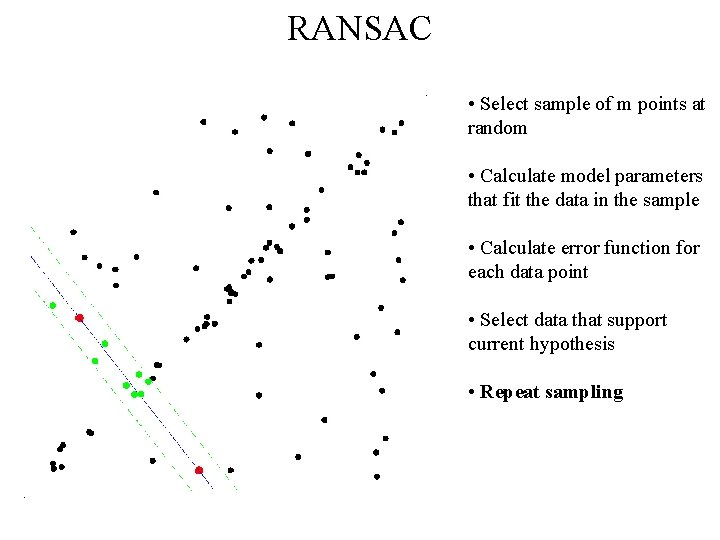RANSAC • Select sample of m points at random • Calculate model parameters that fit the data in the sample • Calculate error function for each data point • Select data that support current hypothesis • Repeat sampling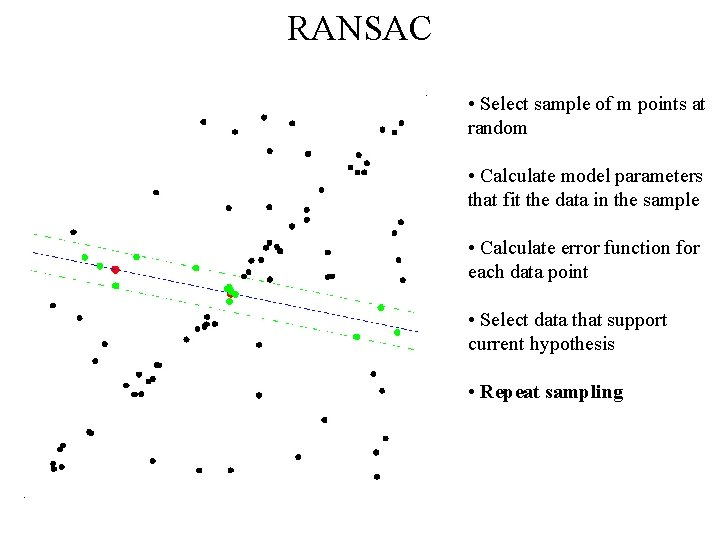RANSAC • Select sample of m points at random • Calculate model parameters that fit the data in the sample • Calculate error function for each data point • Select data that support current hypothesis • Repeat sampling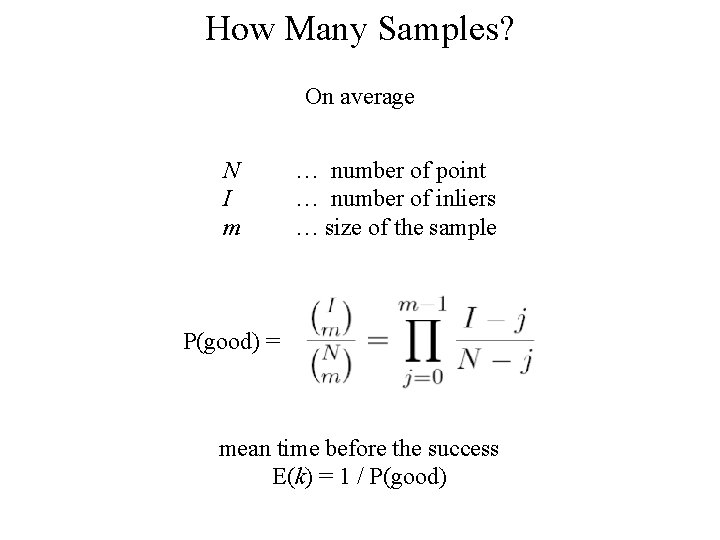How Many Samples? On average N I m … number of point … number of inliers … size of the sample P(good) = mean time before the success E(k) = 1 / P(good)How Many Samples? With confidence pHow Many Samples? With confidence p N I m … number of point … number of inliers … size of the sample P(good) = P(bad) = 1 – P(good) P(bad k times) = (1 – P(good))k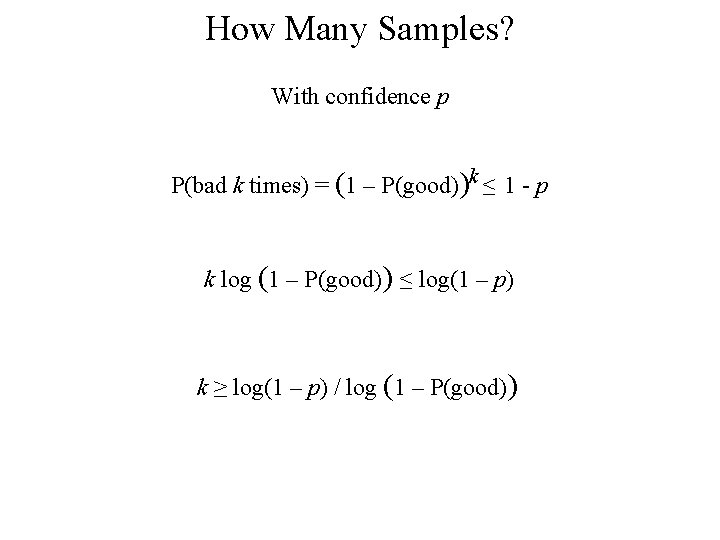How Many Samples? With confidence p P(bad k times) = (1 – P(good))k ≤ 1 - p k log (1 – P(good)) ≤ log(1 – p) k ≥ log(1 – p) / log (1 – P(good))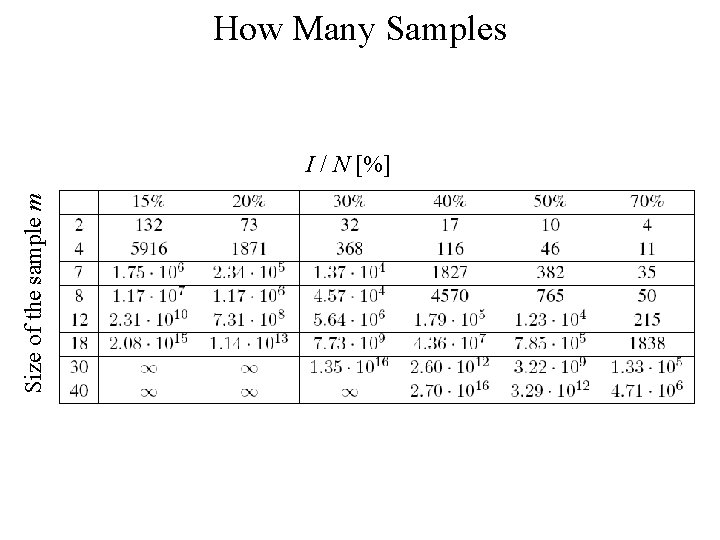How Many Samples Size of the sample m I / N [%]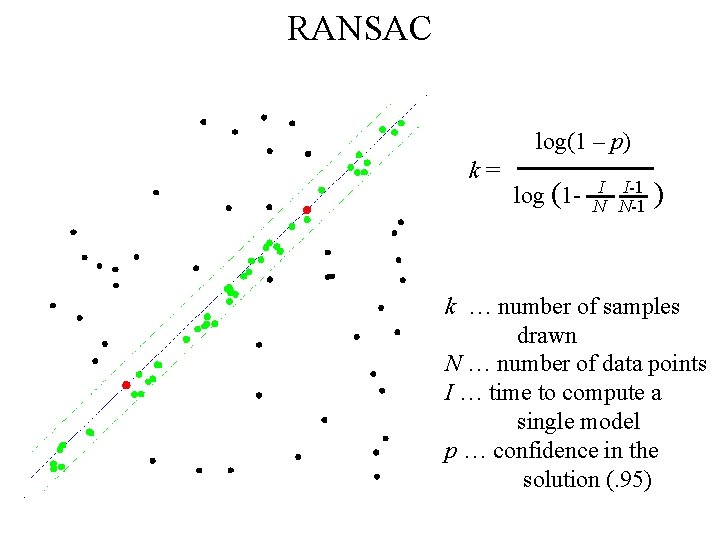RANSAC log(1 – p) k= log (1 - I I-1 N N-1 ) k … number of samples drawn N … number of data points I … time to compute a single model p … confidence in the solution (. 95)RANSAC [Fischler, Bolles ’ 81] In: U = {xi} set of data points, |U| = N function f computes model parameters p given a sample S from U the cost function for a single data point x Out: p* p*, parameters of the model maximizing the cost function k : = 0 Repeat until P{better solution exists} < h (a function of C* and no. of steps k) k : = k + 1 I. Hypothesis (1) select randomly set , sample size (2) compute parameters II. Verification (3) compute cost (4) if C* < Ck then C* : = Ck, p* : = pk endPROSAC – PROgressive SAmple Consensus • Not all correspondences are created equally • Some are better than others • Sample from the best candidates first 1 2 Sample from here 3 4 5 … N-2 N-1 NPROSAC Samples … l-1 l l+1 l+2 … Draw Tl samples from (1 … l) Draw Tl+1 samples from (1 … l+1) Samples from (1 … l) that are not from (1 … l+1) contain Draw Tl+1 - Tl samples of size m-1 and add l+1